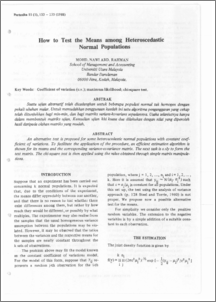# How to Test the Means among Heteroscedastic Normal Populations

## Citation

Abd. Rahman, Mohd Nawi (1988) How to Test the Means among Heteroscedastic Normal Populations. Pertanika, 11 (1). pp. 133-135.

## Abstract

An alternative test is proposed for some heteroscedastic normal populations with constant coefficient of variations. To facilitate the application of the procedure, an efficient estimation algorithm is shown for its means and the corresponding variance-covariance matrix. The next task is only to form the test matrix. The chi-square test is then applied using the value obtained through simple matrix manipulations.Preview
PDF
How_to_Test_the_Means_among_Heteroscedastic.pdfView Item